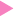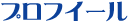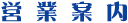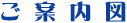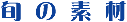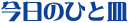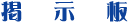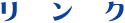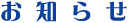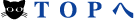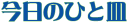\$BBh(B38\$B2s(B 2012. DECEMBER. 10th. \$B\$R\$H\$j\$G\$N\$s\$S\$j%i%s%A(B

\$B!y!y!!%P%C%/%J%s%P!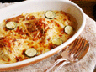\$BBh(B1\$B2s(B 2001 July 15th. \$B!V\$*\$^\$+\$;%i%s%A!WA4KF(B\$BBh(B2\$B2s(B 2001 September 9th. \$B2F\$N=*\$o\$j\$N(B \$B!V\$*\$^\$+\$;%i%s%A!W(B\$BBh(B3\$B2s(B 2001 October 28th. \$B=i=)\$N%i%s%A(B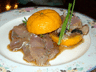\$BBh(B4\$B2s(B 2001 December 15th. \$B:#Lk\$O%Q!<%F%#!<(B!!\$BBh(B5\$B2s(B 2002 January 6th. \$B=i=U\$N%i%s%A(B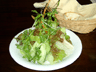\$BBh(B6\$B2s(B 2002 march 26th. \$B=U\$N%i%s%A(B\$BBh(B7\$B2s(B 2002\$B!!(BMay 6th. GW\$BL>;D\$j\$N%i%s%A(B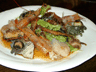\$BBh(B8\$B2s(B 2002 July 27th. \$B%G%#%J!<\$H8@\$&\$h\$j!#!#!#(B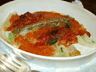\$BBh(B9\$B2s(B 2002 Sep. 23th. \$B=)\$N5YF|(B\$BBh(B10\$B2s(B 2002 Dec. 14th. \$B%/%j%9%^%9A0\$N2:\$d\$+\$JEZMKF|(B\$BBh(B11\$B2s(B 2003 March 8th. \$B=U\$NMr\$NCf\$N%i%s%A(B\$BBh(B12\$B2s(B 2003\$B!!(BMarch 23th. \$B\$*\$@\$d\$+\$J=U\$NF|\$N%i%s%A(B\$BBh(B13\$B2s(B 2003\$B!!(BMay 25th. \$B1+\$NF|\$N%i%s%A(B\$BBh(B14\$B2s(B 2003\$B!!(BAugust 9th. \$BFMA3M<8fHS!*(B\$BBh(B15\$B2s(B 2003 November 29th. \$B;UAvL\A0!*(B \$B\$G\$b\$N\$s\$S\$jM<\$4HS!#(B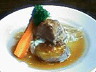\$BBh(B16\$B2s(B 2003 November 30th. \$B:#F|\$b!V3\$G-%i%s%A!W!*(B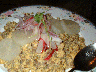\$BBh(B17\$B2s(B 2004 January 3rd. \$B?7=U5-G0\$NM<8fHS!#(B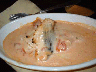\$BBh(B18\$B2s(B 2004 January 11th. \$B:#F|\$bBeBGK?(BK\$B;2>e!*(B\$BBh(B19\$B2s(B 2004 February 19th. \$BAa=U\$N%i%s%A(B\$BBh(B20\$B2s(B 2004 May 3rd. \$B>.4V;H\$\$(BK\$B\$N(B \$B1+\$N(BGW\$B!"2HB2\$N%i%s%A(B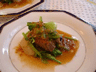\$BBh(B21\$B2s(B 2004 May 23th. \$B=i2F\$N%i%s%A!*(B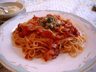\$BBh(B22\$B2s(B 2004 May 26th. \$B=i2FJ?F|\$N%i%s%A(B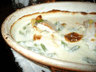\$BBh(B23\$B2s(B 2004 August 25th. \$B2F!"J?F|\$N%i%s%A(B\$BBh(B24\$B2s(B 2004 November 24th. \$B=iE_!"J?F|\$N%i%s%A(B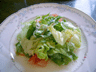\$BBh(B25\$B2s(B 2005 February19th. \$B=i=U\$N%i%s%A!*(B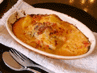\$BBh(B26\$B2s(B 2005 Mar.29th. \$B=U\$O\$d\$C\$Q\$j\$N\$s\$S\$j%i%s%A!*(B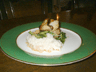\$BBh(B27\$B2s(B 2005 July. 9th. \$BG_1+6u\$N2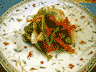\$BBh(B28\$B2s(B 2005 September 11th. \$B5W!9\$N%G%#%J!<(B!!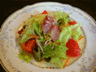\$BBh(B29\$B2s(B 2006 July 18th. \$B5W!9\$N3\$G-8.!*5W!9\$N%i%s%A(B!!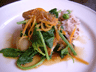\$BBh(B30\$B2s(B 2007. MAY.16th. \$B\$^\$?\$^\$?5W!9\$N3\$G-%i%s%A(B!!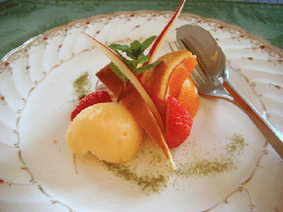\$BBh(B31\$B2s(B 2008. MARCH.12th. \$B=U\$N3\$G-%i%s%A(B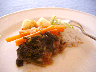\$BBh(B32\$B2s(B 2008. JULY.29th. \$B??2F\$N3\$G-%i%s%A(B\$BBh(B33\$B2s(B 2009. MAY.19th. \$B=i2F\$N%i%s%A!"(B \$B%P!<%9%G%\$%1!<%-IU\$-!*(B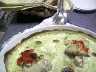\$BBh(B34\$B2s(B 2010. MAY. \$B%7%'%U<+\$i>R2p\$9\$k(B \$B=i2F\$N%i%s%A(B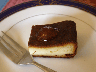\$BBh(B35\$B2s(B 2010. JULY.18th. \$B??2F\$N%i%s%A(B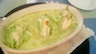\$BBh(B36\$B2s(B 2011. DECEMBER.17th. \$BE_\$N%i%s%A(B\$BBh(B37\$B2s(B 2012. JUNE.17th. \$B=i2F\$N%i%s%A!"%o%\$%sIU\$-(B\$BBh(B38\$B2s(B 2012.DECEMBER.10th. \$B\$R\$H\$j\$G\$N\$s\$S\$j%i%s%A(B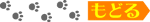\$B!!(Bhealing cuisine \$B3\$G-8.(B\$B!?\$&\$_\$M\$3\$1\$s(B \$BJ!2,8);eEg;T;VK`3)20(B914-2 TEL/FAX 092(328)3117